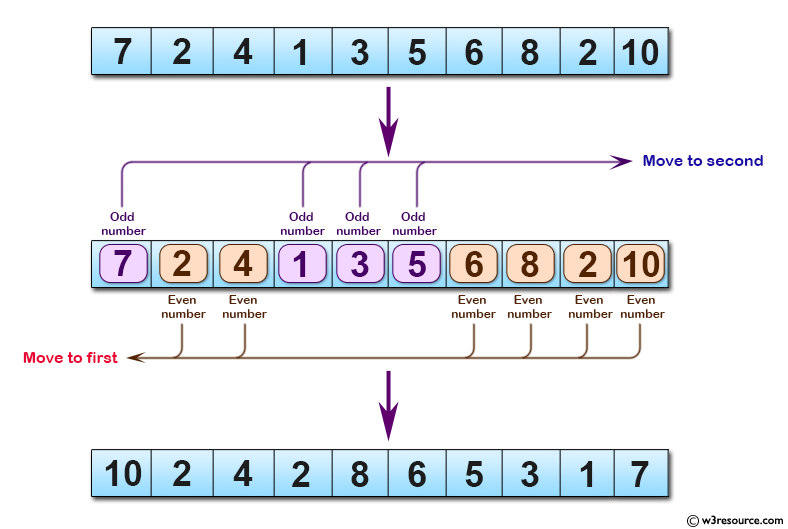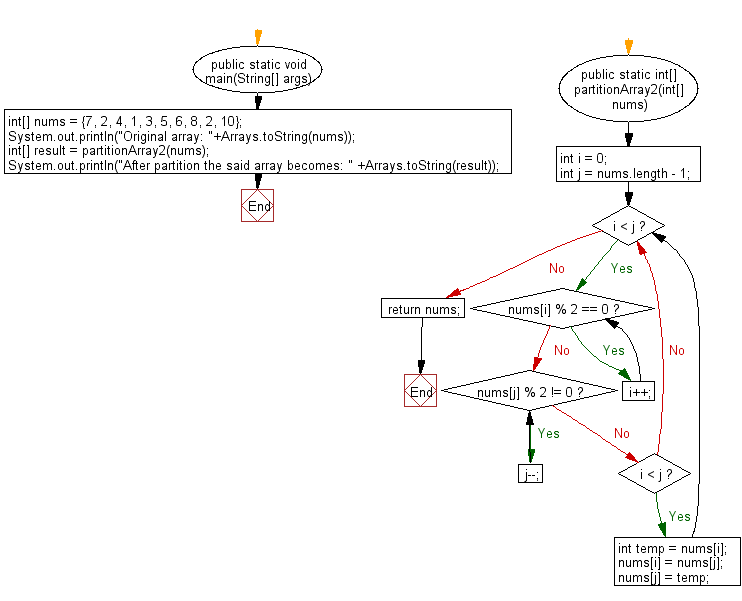﻿ Java programming exercises: Partition an given array of integers into even number first and odd number second - w3resource# Java Exercises: Partition an given array of integers into even number first and odd number second

## Java Basic: Exercise-176 with Solution

Write a Java program to partition an given array of integers into even number first and odd number second.

Original array: [7, 2, 4, 1, 3, 5, 6, 8, 2, 10]
After partition the said array becomes: [10, 2, 4, 2, 8, 6, 5, 3, 1, 7]

Pictorial Presentation:Sample Solution:

Java Code:

``````import java.util.*;

public class Solution {

public static void main(String[] args) {
int[] nums = {7, 2, 4, 1, 3, 5, 6, 8, 2, 10};
System.out.println("Original array: "+Arrays.toString(nums));
int[] result = partitionArray2(nums);
System.out.println("After partition the said array becomes: " +Arrays.toString(result));
}

public static int[] partitionArray2(int[] nums) {
int i = 0;
int j = nums.length - 1;
while (i < j) {
while (nums[i] % 2 == 0) i++;
while (nums[j] % 2 != 0) j--;
if (i < j) {
int temp = nums[i];
nums[i] = nums[j];
nums[j] = temp;
}
}
return nums;
}
}
```
```

Sample Output:

```Original array: [7, 2, 4, 1, 3, 5, 6, 8, 2, 10]
After partition the said array becomes: [10, 2, 4, 2, 8, 6, 5, 3, 1, 7]
```

Flowchart:Java Code Editor:

What is the difficulty level of this exercise?

﻿

## Java: Tips of the Day

Parsing dates:

```import java.io.*;
import java.util.*;
import java.text.*;

String s = "2001/09/23 14:39";

SimpleDateFormat formatter = new SimpleDateFormat ("yyyy/MM/dd H:mm");
Date d = formatter.parse(s, new ParsePosition(0));
```